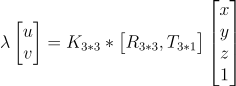# Why do vehichleToImage and worldToImage results not match?

6 views (last 30 days)
cui on 20 Jun 2022
Edited: cui on 29 Jul 2022
As far as I don't fully understand, I think these 2 functions should internally implement the same principle, converting points in the world coordinate system to points on the image pixel coordinate system, without considering distortion, and should satisfy the following equation：% https://ww2.mathworks.cn/help/driving/ref/monocamera.vehicletoimage.html
focalLength = [309.4362 344.2161];
principalPoint = [318.9034 257.5352];
imageSize = [480 640];
intrinsics = cameraIntrinsics(focalLength,principalPoint,imageSize);
height = 2.1798;
pitch = 14;
sensor = monoCamera(intrinsics,height,'Pitch',pitch);
figure
imshow(Ioriginal)
title('Original Image')xyVehicleLoc1 = [10 0];
xyImageLoc1 = vehicleToImage(sensor,xyVehicleLoc1)
xyImageLoc1 = 1×2
318.9034 247.3009
• I then use the worldToImage function to calculate the image points as follows：
R = roty(-pitch);
trans = -R*[0;0;height];
xyImageLoc3 = worldToImage(intrinsics,R',trans',[xyVehicleLoc1,0]) % computer vision toolbox：a departure from conventional practice
xyImageLoc3 = 1×2
1.0e+04 * 1.0726 0.0258
• use above formula
K = intrinsics.IntrinsicMatrix;
xyImageLoc2 = K'*[R,trans]*[xyVehicleLoc1,0,1]';
xyImageLoc2 = xyImageLoc2./(xyImageLoc2(end)) % homogenous coordinates
xyImageLoc2 = 3×1
1.0e+04 * 1.0726 0.0258 0.0001
The results of xyImageLoc1 and xyImageLoc2/3 are different, how can I explain this? How to write the format of worldToImage correctly to convert to vehicleToImage like result?

cui on 20 Jul 2022
Edited: cui on 29 Jul 2022
After trying to experiment, I now have a full grasp of the problem and its internal implementation. I wrote a simple matlab function for C code generation to understand the principle of its monoCamera, vehicleToImage implementation.
function imagePt = vehicleToImgPt(worldPt)%#codegen
focalLength = [309.4362 344.2161];
principalPoint = [318.9034 257.5352];
imageSize = [480 640];
height = 2.1798;
pitch = 14;
%% function
intrinsics = cameraIntrinsics(focalLength,principalPoint,imageSize);
sensor = monoCamera(intrinsics,height,'Pitch',pitch);
imagePt = vehicleToImage(sensor,worldPt);
end
Then generate the main C code belows:main explain is: b_a is [R,t] format array, 3*4 size, rows prioritised. i do experiment by following code, the b_a is same as C code.
% 注意：坐标轴旋转theta角度等价于点旋转-theta角度！
R1 = rotx(pitch)*rotx(90)*rotz(90);% 车辆坐标系到摄像头物理坐标系的转换矩阵。车辆坐标系的坐标轴先绕Z轴旋转-90度，再把坐标轴绕X轴旋转-90度，最后坐标轴再绕X轴旋转-pitch角度。
t1 = -R1*[0;0;height];
b_a = [R1,t1]
ans =
0 -1.0000 0 0
-0.2419 0 -0.9703 2.1151
0.9703 0 -0.2419 0.5273
R1 is the conversion from the vehicle coordinate system to the physical coordinate system of the camera，t1 is corrosponding translation vector.• use formular
imgPt = intrinsics.IntrinsicMatrix'*[R1,t1]*[10;0;0;1];
imgPt = imgPt./imgPt(end)
318.9034
247.3009
1.0000
• use worldToImage function
worldToImage(intrinsics,R1',t1,[10,0,0])
318.9034 247.3009

R2022a

### Community Treasure Hunt

Find the treasures in MATLAB Central and discover how the community can help you!

Start Hunting!